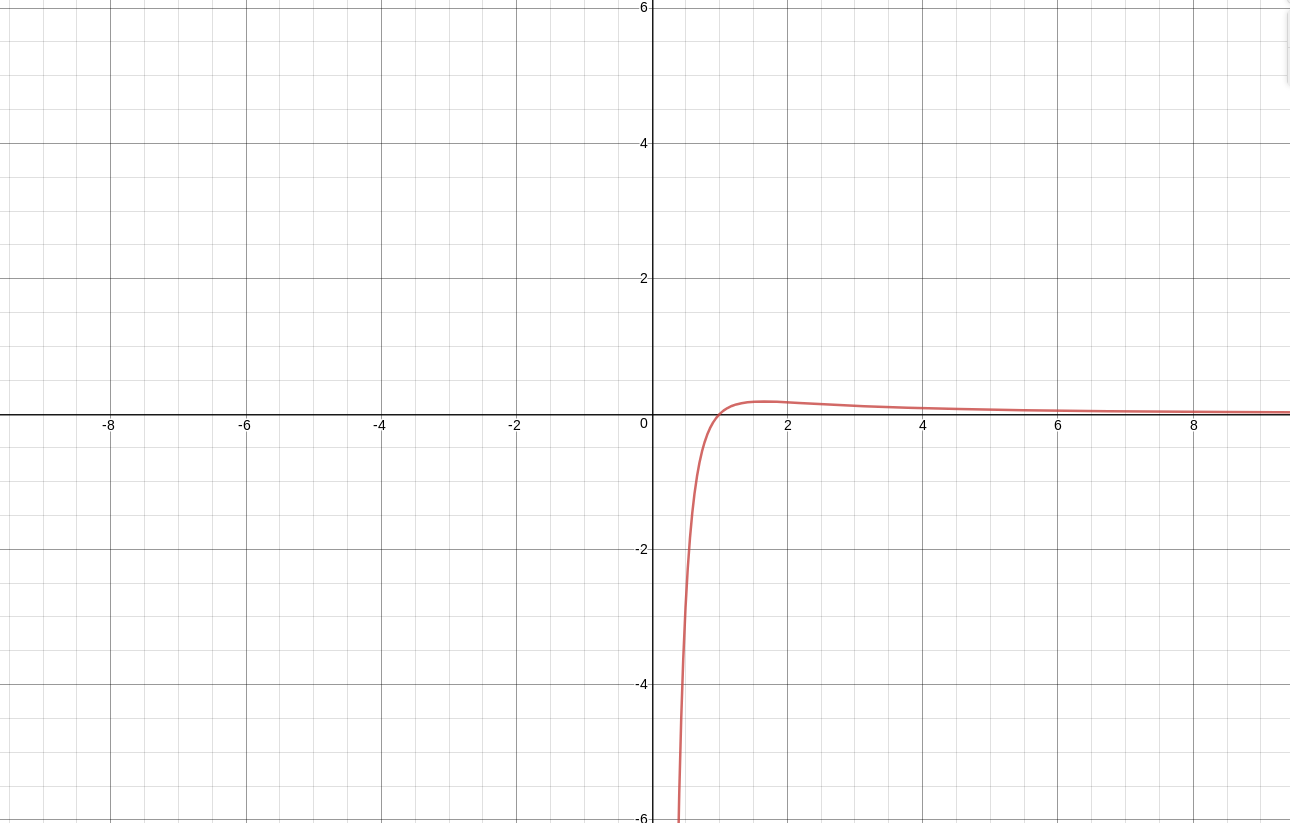# Find maximum among x^(y^2) or y^(x^2) where x and y are given

Given X and Y whose values are greater than 2, the task is to find out which is maximum among

x^(y^2) and y^(x^2)

Consider that either x is greater than y or y is greater than x. So, print 1 if x^(y^2) is greater or 2 if y^(x^2) is greater.

Examples:

```Input: X = 4, Y = 9
Output: 1

Input: X = 4, Y = 3
Output: 2
```

## Recommended: Please try your approach on {IDE} first, before moving on to the solution.

Approach: Assume, then after taking ln on both sides and dividing bywe can get.

Take. This function is monotonically decreasing for.`If x > y, then F(x) < F(y)`

## C++

 `// C++ program to find the greater value ` `#include ` `using` `namespace` `std; ` ` `  `// Function to find maximum ` `bool` `findGreater(``int` `x, ``int` `y) ` `{ ` `    ``// Case 1 ` `    ``if` `(x > y) { ` `       ``return` `false``; ` `    ``} ` ` `  `    ``// Case 2 ` `    ``else` `{ ` `        ``return` `true``; ` `    ``} ` `} ` ` `  `// Driver Code ` `int` `main() ` `{ ` `    ``int` `x = 4; ` `    ``int` `y = 9; ` ` `  `    ``findGreater(x, y) ? cout << ``"1\n"`  `                      ``: cout << ``"2\n"``; ` ` `  `   ``return` `0; ` `} `

## Java

 `// Java program to find ` `// the greater value ` `import` `java.io.*; ` ` `  `class` `GFG  ` `{ ` ` `  `// Function to find maximum ` `static` `boolean` `findGreater(``int` `x,    ` `                           ``int` `y) ` `{ ` `    ``// Case 1 ` `    ``if` `(x > y) ` `    ``{ ` `        ``return` `false``; ` `    ``} ` ` `  `    ``// Case 2 ` `    ``else`  `    ``{ ` `        ``return` `true``; ` `    ``} ` `} ` ` `  `// Driver Code ` `public` `static` `void` `main (String[] args)  ` `{ ` `    ``int` `x = ``4``; ` `    ``int` `y = ``9``; ` `     `  `    ``if``(findGreater(x, y)) ` `    ``System.out.println(``"1"``); ` `    ``else` `    ``System.out.println(``"2"``); ` `} ` `} ` ` `  `// This code is contributed ` `// by inder_verma. `

## Python3

 `# Python3 program to find ` `# the greater value ` ` `  `# Function to find maximum ` `def` `findGreater(x, y): ` `     `  `    ``# Case 1 ` `    ``if` `(x > y):  ` `        ``return` `False``; ` ` `  `    ``# Case 2 ` `    ``else``: ` `        ``return` `True``; ` ` `  `# Driver Code ` `x ``=` `4``; ` `y ``=` `9``; ` `if``(findGreater(x, y)): ` `    ``print``(``"1"``); ` `else``: ` `    ``print``(``"2"``); ` ` `  `# This code is contributed ` `# by mits `

## C#

 `// C# program to find ` `// the greater value ` `using` `System; ` ` `  `class` `GFG  ` `{ ` ` `  `// Function to find maximum ` `static` `bool` `findGreater(``int` `x,  ` `                        ``int` `y) ` `{ ` `    ``// Case 1 ` `    ``if` `(x > y) ` `    ``{ ` `        ``return` `false``; ` `    ``} ` ` `  `    ``// Case 2 ` `    ``else` `    ``{ ` `        ``return` `true``; ` `    ``} ` `} ` ` `  `// Driver Code ` `public` `static` `void` `Main ()  ` `{ ` `    ``int` `x = 4; ` `    ``int` `y = 9; ` `     `  `    ``if``(findGreater(x, y)) ` `        ``Console.WriteLine(``"1"``); ` `    ``else` `        ``Console.WriteLine(``"2"``); ` `} ` `} ` ` `  `// This code is contributed ` `// by inder_verma. `

## PHP

 ` ``\$y``)  ` `    ``{ ` `        ``return` `false; ` `    ``} ` ` `  `    ``// Case 2 ` `    ``else`  `    ``{ ` `        ``return` `true; ` `    ``} ` `} ` ` `  `// Driver Code ` `\$x` `= 4; ` `\$y` `= 9; ` `if``(findGreater(``\$x``, ``\$y``) == true) ` `    ``echo``(``"1\n"``); ` `else` `    ``echo``(``"2\n"``); ` ` `  `// This code is contributed ` `// by inder_verma ` `?> `

Output:

```1
```

My Personal Notes arrow_drop_upCheck out this Author's contributed articles.

If you like GeeksforGeeks and would like to contribute, you can also write an article using contribute.geeksforgeeks.org or mail your article to contribute@geeksforgeeks.org. See your article appearing on the GeeksforGeeks main page and help other Geeks.

Please Improve this article if you find anything incorrect by clicking on the "Improve Article" button below.

Improved By : inderDuMCA, Mithun Kumar

Article Tags :
Practice Tags :

Be the First to upvote.

Please write to us at contribute@geeksforgeeks.org to report any issue with the above content.